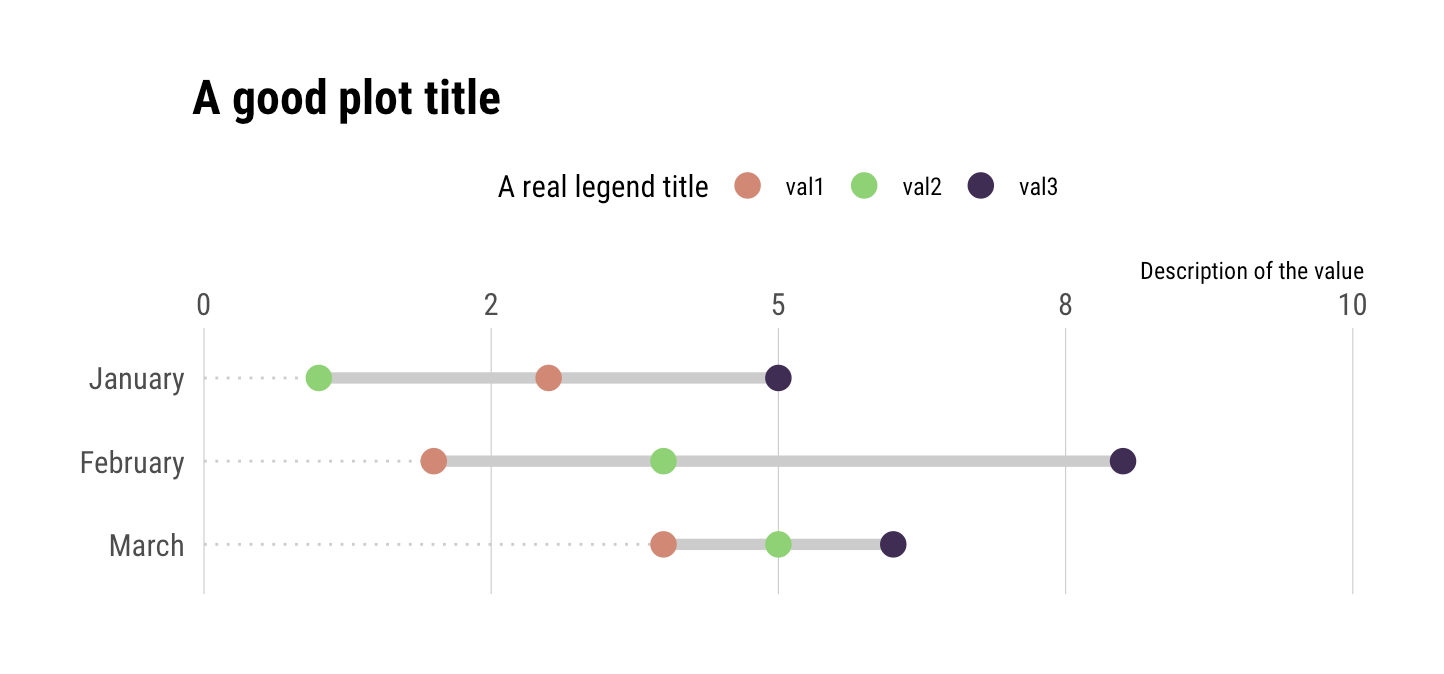# Make Multi-point “dumbbell” Plots in ggplot2

A user of the `{ggalt}` package recently posted a question about how to add points to a `geom_dumbbell()` plot. For now, this is not something you can do with `geom_dumbbell()` but with a bit of data wrangling you can do this in a pretty straightforward manner with just your data and ggplot2. The example below uses 3 values per category but it should scale to `n` values per category (though after a certain `n` you should reconsider the use of a dummbell chart in favour of a more appropriate way to visualize the message you’re trying to convey).

Here’s the setup:

``````library(hrbrthemes)
library(tidyverse)

tibble(
val1 = c(3, 2, 4),
val2 = c(1, 4, 5),
val3 = c(5, 8, 6),
cat = factor(month.name[1:3], levels = rev(month.name[1:3]))
) -> xdf
``````

Three values per category. The approach is pretty straightforward:

• reshape the data frame & get min value so you can draw an eye-tracking line (this is one geom)
• reshape the data frame & get min/max category values so you can draw the segment (this is another geom)
• reshape the data frame & plot the points

I’ve put ^^ notes near each ggplot2 geom:

``````ggplot() +
# reshape the data frame & get min value so you can draw an eye-tracking line (this is one geom)
geom_segment(
data = gather(xdf, measure, val, -cat) %>%
group_by(cat) %>%
top_n(-1) %>%
slice(1) %>%
ungroup(),
aes(x = 0, xend = val, y = cat, yend = cat),
linetype = "dotted", size = 0.5, color = "gray80"
) +
# reshape the data frame & get min/max category values so you can draw the segment (this is another geom)
geom_segment(
data = gather(xdf, measure, val, -cat) %>%
group_by(cat) %>%
summarise(start = range(val), end = range(val)) %>%
ungroup(),
aes(x = start, xend = end, y = cat, yend = cat),
color = "gray80", size = 2
) +
# reshape the data frame & plot the points
geom_point(
data = gather(xdf, measure, value, -cat),
aes(value, cat, color = measure),
size = 4
) +
# i just extended the scale a bit + put axis on top; choose aesthetics that work
# for you
scale_x_comma(position = "top", limits = c(0, 10)) +
scale_color_ipsum(name = "A real legend title") +
labs(
x = "Description of the value", y = NULL,
title = "A good plot title"
) +
theme_ipsum_rc(grid = "X") +
theme(legend.position = "top")
``````

And, here’s the result:Amazon Author Page

## 4 Comments →Make Multi-point “dumbbell” Plots in ggplot2

1.numerategeek

thanks very much for this. very helpful and timely – I was thinking of using dumbbell charts to visualize some of my data. thank you!

This site uses Akismet to reduce spam. Learn how your comment data is processed.